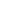﻿ Determination of Temperature Distribution in a Cylindrical Nuclear Fuel Rod – a Mathematical Approach - Volume 1 Number 5 (Dec. 2010) - IJIMT
﻿IJIMT 2010 Vol.1(5):464-465  ISSN: 2010-0248
DOI: 10.7763/IJIMT.2010.V1.81

# Determination of Temperature Distribution in a Cylindrical Nuclear Fuel Rod – a Mathematical Approach

K. M. Pandey and M. Mahesh

Abstract—The steady state temperature distribution in a nuclear fuel rod has been discussed in this paper. Energy is released by fission within the nuclear fuel rod. This energy is then transferred by heat conduction to the surface of the fuel rod. From the surface of the nuclear fuel rod heat is transferred by convection to the coolant (water), which passes from the core to the external heat exchangers in which steam is generated to operate on a power cycle. The fuel elements are usually long cylindrical rods (which has been assumed in this paper) or rectangular plates of uranium (or thorium). The non-uniform heat generation has been employed in this paper. A cylindrical geometry with some assumptions has been considered. The geometry of the nuclear fuel rod is studied initially and then the symmetry considerations are carried out. The governing differential equation for the steady state condition with internal heat generation is rolled on. The numerical method of solution is used extensively in practical applications to determine the temperature distribution and heat flow in solids having complicated geometries, boundary conditions, and temperature-dependent thermal properties. A number of numerical schemes are available. In this paper the finite-difference method is used. The problem is then discretized and the numerical method of solutions is played out using finite difference method. Then the nature of the non-dimensional temperature distribution is observed and studied.

Index Terms—Temperature distribution, Nuclear fuel rod, Steady-state Heat conduction, Internal heat generation, Finite difference method.

[PDF]

Cite: K. M. Pandey and M. Mahesh, " Determination of Temperature Distribution in a Cylindrical Nuclear Fuel Rod – a Mathematical Approach," International Journal of Innovation, Management and Technology vol. 1, no. 5, pp. 464-465, 2010.

Copyright © 2010-2018. International Journal of Innovation, Management and Technology. All rights reserved.
E-mail: ijimt@ejournal.net